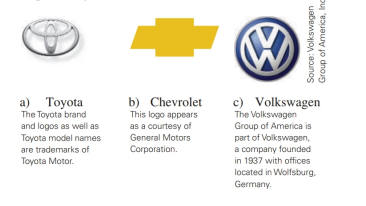Chapter 2.6, Problem 27E### Elementary Geometry for College St...

6th Edition
Daniel C. Alexander + 1 other
ISBN: 9781285195698

#### Solutions

Chapter
Section### Elementary Geometry for College St...

6th Edition
Daniel C. Alexander + 1 other
ISBN: 9781285195698
Textbook Problem
8 views

# Describe the type(s) of symmetry displayed by each of thesecorporate logos.To determine

a)

The type(s) of symmetry displayed by the given logo

Explanation

Given:

The given logo is,

Figure (1)

Definition Used:

(1) If for every point A on the figure there is a second point A on the figure for which line l is the perpendicular bisector of AA¯, then the figure has symmetry with respect to line l.

(2) If for every point A on the figure there is a second point A on the figure for which point P is the midpoint of AA¯, then the figure has symmetry with respect to point P

To determine

b)

The type(s) of symmetry displayed by the given logo

To determine

c)

The type(s) of symmetry displayed by the given logo

### Still sussing out bartleby?

Check out a sample textbook solution.

See a sample solution

#### The Solution to Your Study Problems

Bartleby provides explanations to thousands of textbook problems written by our experts, many with advanced degrees!

Get Started

#### In Exercises 7-10, find the slope of the line shown in each figure. 10.

Applied Calculus for the Managerial, Life, and Social Sciences: A Brief Approach

#### What are the two requirements for a random sample?

Statistics for The Behavioral Sciences (MindTap Course List)

#### Polar coordinates of the point with rectangular coordinates (5, 5) are: (25, 0)

Study Guide for Stewart's Single Variable Calculus: Early Transcendentals, 8th